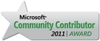• ### Re: SQL Update column based on count

Hello Gordon,

Your requirement is very interesting for me.

I have not worked on such a case, I will provide a solution for this. I'm not sure if it is the best one but the solution seems to be working quite well according to me.

I'll soon try to enhance the solution at Update Table Data for Uniform Distribution in SQL of course if the uniform distribution is the correct name on this case

Please check below SQL Update CTE command

```;with summary as (
SELECT distinct
COUNT(*) Over (Partition By 1) Cnt,
RevId,
COUNT(RevId) Over (Partition By RevId) RevCnt
FROM MyTable
), todo as (
select
Cnt,
Cnt / (count(*) over (partition by 1)) Average,
RevId, RevCnt
from summary
), joint as (
select
id,
MyTable.RevId,
orderno = ROW_NUMBER() over (partition by MyTable.RevId order by id),
Cnt,
Average,
RevCnt,
RevCnt - Average as forupdate
from MyTable
inner join todo on MyTable.RevId = todo.RevId
), upd as (
select *
from joint
where RevCnt < Average and orderno <= (forupdate * -1)
union all
select *
from joint
where RevCnt > Average and orderno <= forupdate
), final as (
select
id, RevId,
case when forupdate > 0 then 1 else -1 end as overAvg,
rn = ROW_NUMBER() over (partition by (case when forupdate > 0 then 1 else -1 end) order by id)
from upd
)
update MyTable
set
RevId = ISNULL(n.RevId,p.RevId)
from MyTable t
inner join final p on p.id = t.id
left join final n on p.rn = n.rn and n.overAvg = -1
where p.overAvg = 1
```

I strongly suggest you to test the script on different types of data distribution before you use it.

I used multiple CTE expressions before update statement is formed. Also many SQL functions like Row_Number() with Partition By clause and SQL Aggregate functions like Count() with Partition By clause, etc.

I hope it helps you for solution,

Hi Gordon,

I tested the above code block with different data sets, in some cases you need to execute the code twice. First execution minimizes the difference between counts of different categorized values, second will make them near to equal

T-SQL programming SQL Server Tutorials
SQL Server 2017